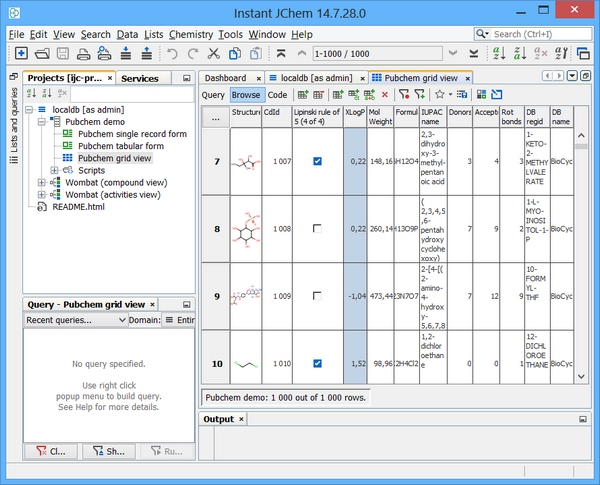# Triggers and sequences: Oracle

The following four sections of this page explain how to complete the following tasks :
1. Administer a table in Oracle using Instant JChem.
2. Add a text field and populate it with a character based unique key.
3. Define an approach to derive a BOOLEAN data item "Lipinski rule of 5" for new rows based upon chemical terms, implemented as a "BEFORE INSERT" trigger.
4. Define an approach to synchronise columns for which the source data is subsequently updated, implemented as a "BEFORE UPDATE" trigger. This operation is required for example after a re-standardizaion operation is applied on a given JChem table.

It is hoped that these examples can be used as templates for further bespoke index based on Chemical terms or other calculated columns. In the case of Oracle "instant client" is available for download and will contain the client side SQLPLUS tool which can connect to the Oracle instance and issue commands.

Alternatively the IJC database explorer will negate the need for SQLPLUS and will allow administration tasks as explained here: Using the database explorer

# Administer Oracle table using IJC

First, we need to administer a JChem table. One method to do this is via Instant JChem (IJC) Desktop application. Assuming we have an existing Oracle connection / schema established in IJC then we can add a new JChem table easily. Right click on your schema and choose "new structure entity table". At the entity type drop down choose "New structure entity (using JChemBase table)". Assuming we accept the defaults - we should see the window below and the subsequent examples assume a table name of "STRUCTURES"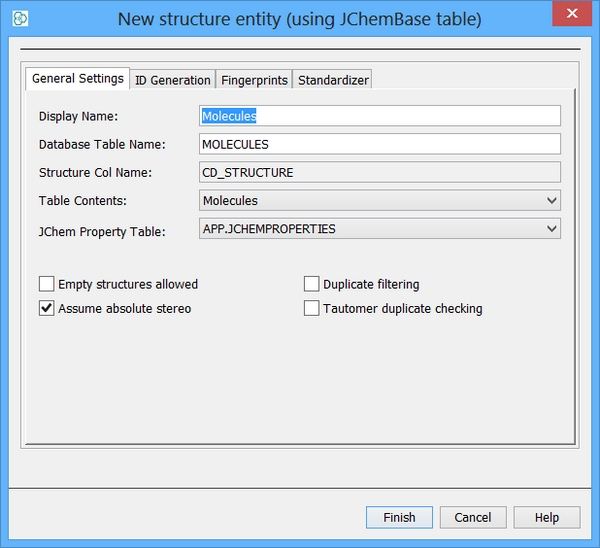# Character based Unique key generation

A JChem table already has a build in unique integer key (CD_ID), however some organisations prefer to have a character based identifier since this can aid internal communication of structures. In order to create a character based sequence the integer based sequence is embedded in a trigger and prefixed with an appropriate string in this case "mol-" is used. If this is a "private" database then the Chemist initials (or lab notebook reference) might be suitable or perhaps a corporate identifier string is suitable if working with the central data repository.

An additional text based column is now required to store the character based key and this can be created using IJC. Right click on your table and choose "Edit data tree" Left click on "New standard field" and choose "Text field". You can also edit your display name and column name. In this case the column is named "TARGET_KEY". The Text field maps directly to a column of type VARCHAR2 in Oracle.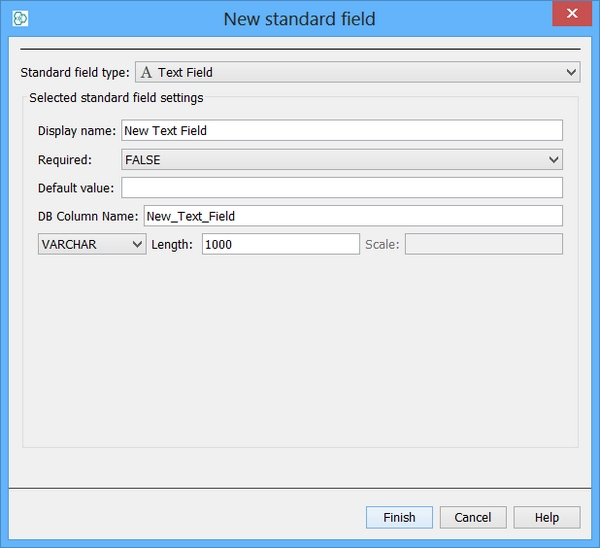In order to create an integer based sequence in Oracle the following syntax is required and can be executed using SQLPLUS client which Oracle provides.

`CREATE`` ``SEQUENCE`` seq_structures START ``WITH`` 1 INCREMENT ``BY`` 1;`

The sequence methods "nextval" and "currval" can be used to obtain next and current numbers from an existing sequence object. Since the sequence is integer based, a character coversion function is required.

`CREATE`` ``OR`` ``REPLACE`` ``TRIGGER`` structures_key_trg BEFORE ``INSERT`` ``ON`` structures `
`FOR`` EACH ROW ``BEGIN`` :NEW.target_key := ``'mol-'`` || TO_CHAR (seq_structures.nextval); ``END``;/`

To fire the trigger we should simply insert data into the table and for every row the action will occur. IJC will be completing the insert (during data import) so you should not need to execute this syntax but your trigger will fire as a result of this action, if you should wish to test

`INSERT`` ``INTO`` structures (cd_id) ``VALUES`` (``'1'``);`

# Derived data item : Lipinski rule of 5 filter (New Inserts)

In order to create derivations from existing data an individual or set of triggers can be used. In this example a single drug like filter column "Lipinski_5" is created and populated for any rows that are added to a JChem table. The IJC boolean type actually maps to a NUMBER type in Oracle.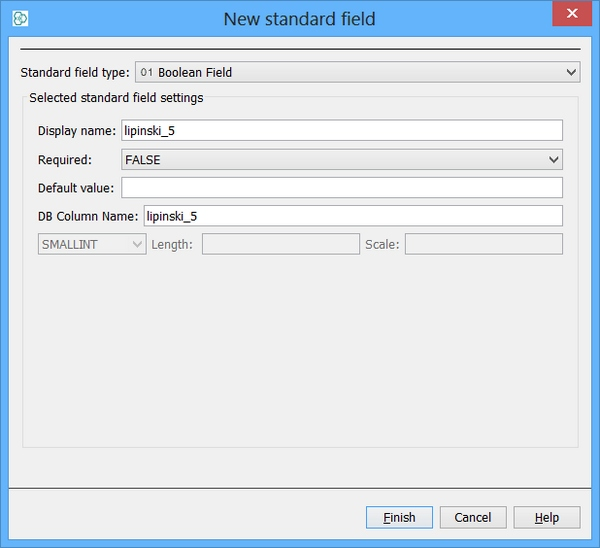A pre-requisite for this field is the existence of the necessary chemical terms columns it is derived from (at compile time). Also it is assumed they will be populated and not NULL and if created prior to a data import they will be populated. Several filter definitions already exist but the use of triggers provides an alternative option to avoid some repeat calculation and of course create new bespoke definitions. The Lipinski defintion example here is taken from the following reference but has been slighty modified to include the value in each clause :

Reference : Chemoinformatics (Wiley), Page 607, Gasteiger J.

1. molecular weight <= 500 g / mol
2. a calculated log p <= 5
3. H-bond donors <= 5
4. H-bond acceptors <= 10
5. rotatable bond count <= 10

As such the following Chemical terms fields are selected for addition to the table and are populated on data import.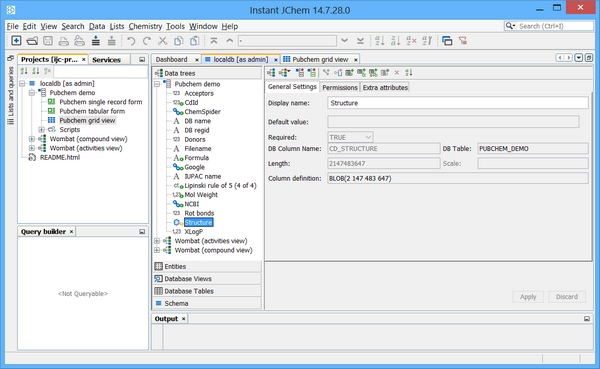The Trigger can now be created as follows:

`CREATE`` ``OR`` ``REPLACE`` ``TRIGGER`` structures_lipinski5_instrg`
`BEFORE ``INSERT`` ``ON`` structures ``FOR`` EACH ROW ``BEGIN`` :NEW.lipinski_5 := 0;`
`IF (:NEW.cd_moleight <= 500 ``AND`` :NEW.logp <= 5 ``AND`` :NEW.h_bond_donors <= 5 ``AND`` :NEW.h_bond_acceptors <= 10 ``AND`` :NEW.rotatable_bonds <= 10) ``THEN`
`:NEW.lipinski_5 := 1;`
`END`` IF;`
`EXCEPTION ``WHEN`` OTHERS ``THEN`` RAISE;`
`END``;/ `

# Derived data time: Lipinsi rule of 5 filter (After table re-standartization or structure edit)

If the standardization rules for a given table are modified or a chemical structure edited then potentially the chemical terms might change also. Any index based on these columns will have no update mechanism defined. As such, one should also consider an after update trigger to re-calculate derived fields after a re-standardization event. In Oracle this can be made to fire only when the relevant columns are updated. The Trigger can now be created as follows ...

`CREATE`` ``OR`` ``REPLACE`` ``TRIGGER`` structures_lipinski5_updtrg`
`BEFORE ``UPDATE`` ``OF`` cd_molweight,logp,h_bond_donors,h_bond_acceptors,rotatable_bonds`
`ON`` structures ``FOR`` EACH ROW ``BEGIN`` :NEW.lipinski_5 := 0;`
`IF (:NEW.cd_moleight <= 500 ``AND`` :NEW.logp <= 5 ``AND`` :NEW.h_bond_donors <= 5 ``AND`` :NEW.h_bond_acceptors <= 10 ``AND`` :NEW.rotatable_bonds <= 10) ``THEN`
`:NEW.lipinski_5 := 1;`
`END`` IF;`
`EXCEPTION ``WHEN`` OTHERS ``THEN`` RAISE;`
`END``;/`

The end result is a drug like filter and less time screening unnecessary molecules ...